## ISC Physics Previous Year Question Paper 2011 Solved for Class 12

Maximum Marks: 70
Time allowed: 3 hours

• Candidates are allowed additional 15 minutes for only reading the paper. They must NOT start writing during this time
• Answer all questions in Part I and six questions from Part II, choosing two questions from each of the Sections A, B and C.
• All working, including rough work, should be done on the same sheet as, and adjacent to, the rest of the answer.
• The intended marks for questions or parts of questions are given in brackets [ ].
• Material to be supplied: Log tables including Trigonometric functions
• A list of useful physical constants is given at the end of this paper.

Part-I

Question 1.
A Choose the correct alternative A, B, C or D for each of the questions given below : 
(i) Two point charges (+e) and (-e) are kept inside a large metallic cube without touching its sides. Electric flux emerging out of the cube is:
(A) $$\frac{e}{\epsilon_{o}}$$
(B) $$\frac{-e}{\epsilon_{o}}$$
(C) Zero
(D) $$\frac{2 e}{\epsilon_{o}}$$

(ii) In current electricity, Ohm’s law is obeyed by all:
(A) solids
(B) metals
(C) liquids
(D) gases

(iii) When a charged particle is projected perpendicular to a uniform magnetic field, it describes a circular path in which:
(A) its speed remains constant.
(B) its velocity remains constant.
(C) its momentum remains constant.
(D) its kinetic energy increases.

(iv) Refractive index of a transparent material is:
(A) same for all colors.
(B) maximum for violet color.
(C) minimum for violet color.
(D) maximum for red color.

(v) Which one of the following graphs in figure below represents variation of de Broglie wavelength (λ) of a particle having linear momentum p:
(A)(B)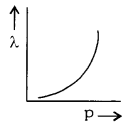(C)(D)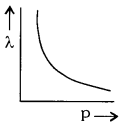B. Answer all questions briefly and to the point: 
(i) How much work is done in taking an electron around a nucleus in a circular path ?

(ii) A 10 m long potentiometer wire carries a steady current. A standard cell of emf 1.018 V is balanced against a length of 254.5 cm of the wire. What is the potential gradient across the potentiometer wire ?

(iii) Name any one instrument which works on the principle of Tangent law in magnetism.

(iv) An inductor L and a resistor R are connected in series to a battery, through a key/switch. Show graphically, how current decreases with time when the key/switch is opened.

(v) An ideal inductor does not consume any power even though both V and I are non zero. Explain in brief.

(vi) In Fraunhofer’s single slit diffraction experiment, how does semi-angular width θ of the central bright fringe depend on slit width ‘a’ ?

(vii) State one use of a polaroid.

(viii) A ray of light LM incident normally on the surface AC of an isosceles right angled prism ABC (where AB = BC) emerges along PQ, parallel to LM, as shown in figure below: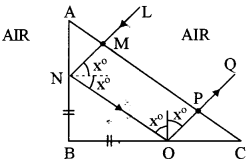What can you say about refractive index µ. of the material of the prism ?

(ix) State one condition for obtaining a sustained interference of light.

(x) State any one postulate of Huygens’s wave theory.

(xi) How can an n – type semiconductor be obtained from a pure crystal of germanium ?

(xii) In the following nuclear reaction
$$_{6}^{11} \mathrm{C} \rightarrow_{5}^{11} \mathrm{B}+_{1}^{0} \mathrm{e}+\mathrm{X}$$
what does X stand for ?

(xiii) In photoelectric effect, what is meant by the term ‘threshold frequency’ ?

(xiv) Find angular momentum of an electron when it is in the second Bohr orbit of hydrogen atom,

(xv) What is the symbol of a NOT gate ?
A. (i) C, since the net charge enclosed is zero.

(ii) B, all metals obey Ohm’s law.

(iii) A, because magnetic field does cause any change in K.E. of the particle,

(iv) B, as wavelength decreases, refractive index increases.

(v) $$\mathrm{D}, \text { as } \lambda=\frac{h}{p} \text { i.e., } \lambda \propto \frac{1}{p}$$

B. (i) No work will be done in moving the electron in a circular path around the nucleus because force and displacement are mutually perpendicular; hence $$\overline{\mathbf{F}} \cdot \overline{d s}=0$$

(ii) If a length ‘l’ of the potentiometer wire balances a cell of emf ‘E’, then E = kl, where ‘k’ is the potential gradient.
Here,
$$\begin{array}{l}{\mathrm{E}=1.018 \mathrm{V} \text { and } l=254.5 \mathrm{cm}} \\ {k=\frac{\mathrm{E}}{l}=\frac{1.018 \mathrm{V}}{254.5 \mathrm{cm}}=4 \times 10^{-3} \mathrm{V} / \mathrm{cm}}\end{array}$$

(iii) One instrument which works on the principle of tangent law in magnetism is a tangent galvanometer.

(iv) The required graph of the variation of current with time is as shown :(vii) Polaroids are used:
(i) in windowpanes of the aeroplanes.
(ii) The sunglasses are made up of Polaroid sheets to avoid glare of sunlight reflected from bright surfaces.

(viii) The angle marked ×° in the fig. is 45°. Since the LN suffers total internal reflection, the critical angle C must be less than 45°.(ix) The two sources of light must be coherent. Such sources are derived from the same source.

(x) One postulate of Huygens’s wave theory is : Every point on the wave front acts as a new wave-source from which fresh wave originates. These waves are called ‘secondary wavelets.’

(xi) A semiconductor is obtained from a pure germanium crystal by doping it with a pentavalent impurity like arsenic, antimony.

(xii) X stands for γ-rays. Conservation law gives both its mass number and atomic number to be zero.

(xiii) The lowest frequency of light which can emit photo electrons from a material is called ‘threshold frequency’ or ‘cut-off frequency’ of that material.

(xiv) Angular momentum of an electron revolving around the nucleus in an hydrogen atom is quantised and is given by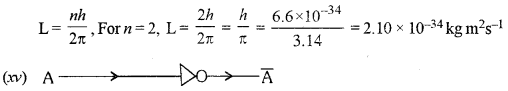Part-II
Answer six questions in this part, choosing two questions from each of the Sections A, B and C

Question 2.
(a) Obtain an expression for intensity’ of electric field in end on position, i. e., axial position of an electric dipole. 

(b) Three capacitors each of capacitance C are connected in series. Their equivalent capacitance is Cs. The same three capacitors are now connected in parallel. Their equivalent capacitance becomes Cp. Find the ratio $$\left(\frac{\mathrm{C}_{\mathrm{p}}}{\mathrm{C}_{\mathrm{s}}}\right)$$ (Working must be shown) 

(c) A galvanometer with a resistance of 75 Ω produces a full scale deflection with a current of 5 mA. How can this galvanometer be converted into an ammeter which has a range of 0 – 5 A ? 
(a) Intensity of electric field in end on position i.e., axial position of an electric dipole: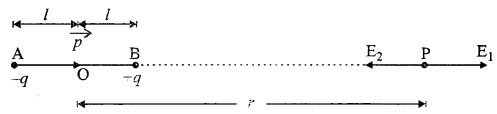Let AB be an electric dipole made up of two charges of -q and +q coulomb having a small distance 2l meter between them situated in vacuum. Let ‘P’ be a point on the axis at a distance V meter from the mid-point ‘0: of the dipole.
We have to determine the intensity at ‘P’.
Let E1 and E2 be the magnitudes of the intensities of the electric dipole at ‘P’ due to the charges + q and -qof the dipole respectively. The distance of the point P from the charge +q is (r – l) and that from the charge – q is (r + l). Therefore,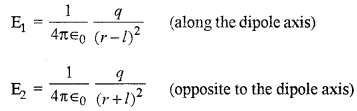Question 3.
(a) In the circuit shown below in figure, E1 and E2 are batteries having emfs 4.0 V and 3.5 V respectively and internal resistance 1 Ω and 2 Ω. respectively. Using Kirchhoff’s laws, calculate currents : I1 I2 and I3.(b) Show with the help of a labelled graph, how thermo emf ‘e’ developed by a thermocouple varies with θ, the temperature difference between the two junctions. On the graph, mark neutral temperature as θN and temperature of inversion as θI. 

(c) (i) What is meant by a paramagnetic substance ? State Curie’s law.
(ii) What is the value of magnetic susceptibility of Aluminium if its relative permeability is 1.000022?
(a) The distribution of current is as shown in the figure :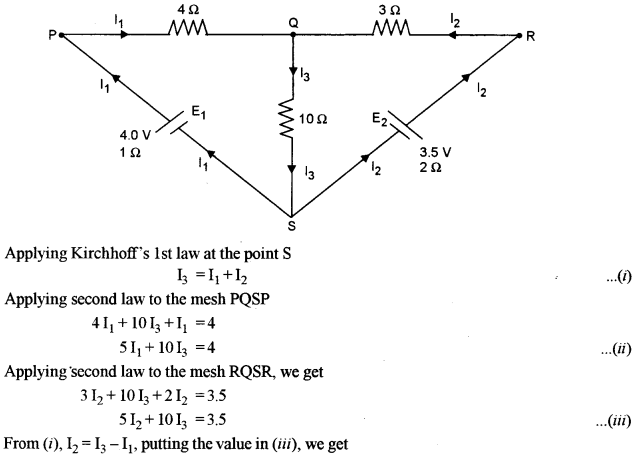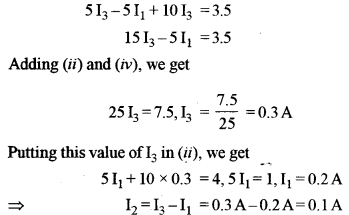(b) Variation of thermo emf ‘e’ with ‘θ’ is as shown below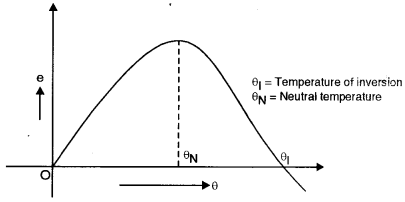(c) (i) Para magnetic substances : These substances are feebly attracted by a magnet. When placed in a magnetizing field, they are feebly magnetized in the direction of the field, that is, the magnetization I in para magnetic materials is weak, but in the same direction as the magnetizing field, thus, they have a small positive susceptibility Xm .
Curie’s law: States the susceptibility ‘ Xm‘ of a para magnetic substance varies inversely as the kelvin-temperature of the substance, i.e..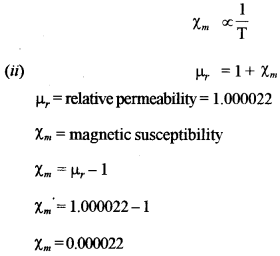Question 4.
(a) Using Ampere’s circuital law or Biot and Savart’s law, show that magnetic flux density ‘B’ at a point ‘P’ at a perpendicular distance ‘a’ from a long current carrying conductor is given by:
$$B=\left(\frac{\mu_{0}}{4 \pi}\right) \frac{2 I}{a}$$
(Statement of the laws – not required)

(b) A current of 4 A flows in a coil when it is connected to a 12 V dc source. When the same coil is connected to an ac source (12 V, 8 Hz), a current of 2.4 A flows in the coil. Calculate coefficient of self inductance (L) of the coil. 

(c) How much force per unit length acts on a long current carrying conductor X due to a current flowing through another similar conductor Y, kept parallel to it in vacuum ? 
Use this equation to define an Ampere, the fundamental unit of current.
(a) Consider a long straight conductor carrying a current I amp. It is desired to find the magnetic flux density at P at a distance a from the conductor. The lines of force will be circular with the center on the conductor.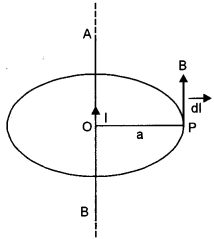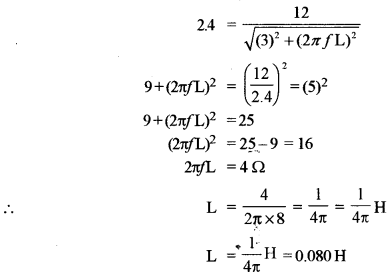(c) The force per unit length of a conductor X carrying current due to a current I2 flowing in another conductor Y placed parallel to X is given by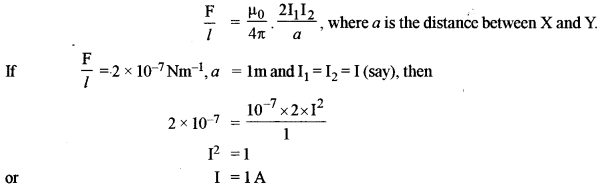Ampere : One ampere of current is that much current which when flowing through each of two infinitely long parallel conductors held 1m apart in vacuum results in a force of 2 x 10-7 Nm-1 on each of the conductor.

Section-B

Question 5.
(a) (i) Arrange all the seven types of electro-magnetic radiations in increasing order of their frequencies. (You must begin with a radiation with lowest frequency and end with the one having the highest frequency.) 
(ii) State how electric vector $$\overrightarrow{\mathrm{E}}$$, magnetic vector $$\overrightarrow{\mathrm{B}}$$ and velocity vector $$\overrightarrow{\mathrm{C}}$$ are oriented in an electromagnetic wave.

(b) In Young’s double slit experiment, using monochromatic light L1 of wavelength 700 nm, 10th bright fringe was obtained at a certain point P on a screen. Which bright fringe will be obtained at the same point P if monochromatic light of wavelength 500 nm is used in place of L1. (No other alterations were made in the experimental set up.) 

(c) A certain monochromatic light travelling in air is incident on a glass plate at a polarizing angle. Angle of refraction in glass is found to be 32°. Calculate : 
(i) the polarizing angle;
(ii) refractive index of glass.
(a) (i) Long waves → Radio waves → Microwaves, Infrared radiation → Visible light → Ultraviolet radiation → X-rays → Gamma rays.
(ii) The electric vector $$\overrightarrow{\mathrm{E}}$$, magnetic vector $$\overrightarrow{\mathrm{B}}$$ and velocity vector $$\overrightarrow{\mathrm{C}}$$ are mutually perpendicular.Question 6.
(a) Calculate angle of minimum deviation (δm) for a regular glass prism. (Refractive index of glass = 16) 

(b) Obtain an expression for refraction at a single convex spherical surface, i. e., the relation between μ1 (rarer medium), μ2 (denser medium), the object distance u, image distance v and radius of curvature R. 

(c) Where should an object be kept on the principal axis of a convex lens of focal length 20 cm, in order to get an image, which is double the size of the object ?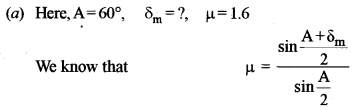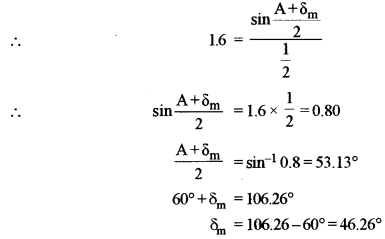(b) Let O be a point object in the rarer medium of refractiive index μ1 and lying on the principal axis. The image of this object. O is formed by refraction at the convex spherical surface of radius at the point I into the medium B of refractive index μ2 of curvature as shown in the fig. The convex surface has a small aperture and the angles of incidence z and refraction r are small.
Let ∠AOP = α, ∠AIP = β and ∠ACP = γ
From A, draw AN perpendicular to principal axis. For refraction at the point A, by Snell’s law, we have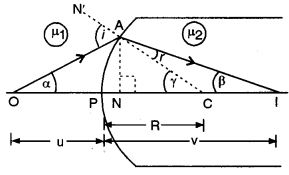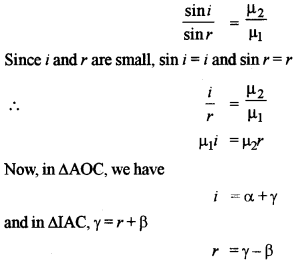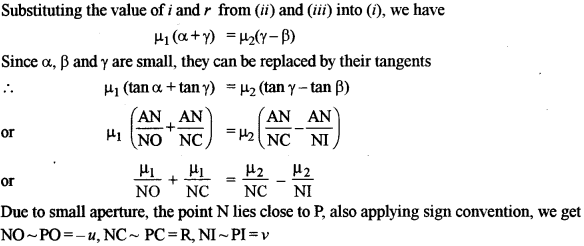Question 7.
(a) (i) What is the use of a spectrometer ? 
(ii) In a spectrometer, what is the function of:

• Collimator ?
• Telescope ?

(b) Draw a labelled diagram of an image formed by a compound microscope with image at least distance of distinct vision. 

(c) An astronomical telescope consists of two thin convex lenses having focal lengths of 140 cm and 5 cm. The telescope is adjusted to be in normal adjustment. 
(i) What is the angular magnification, 7 . e., magnifying power of the telescope in this set up ?
(ii) What is the distance between the two lenses equal to ?
(a) (i) Spectrometer is used for measurement of dispersive powers, wavelength of spectral lines, the refractive indices of solids and liquids.
(ii) (1) Collimator’s function is to alter the distance between the slit and the lens by rack and pinion arrangement. The slit is adjusted to be at the focus of the collimating lens so as to get a parallel beam of light.
(2) Telescope function is to adjust the distance between lens and the cross-wires by rack and pinion arrangement, while that between cross-wires and eye piece by hand. The objective of the telescope forms a real image of the slit. This image is then magnified by the eyepiece.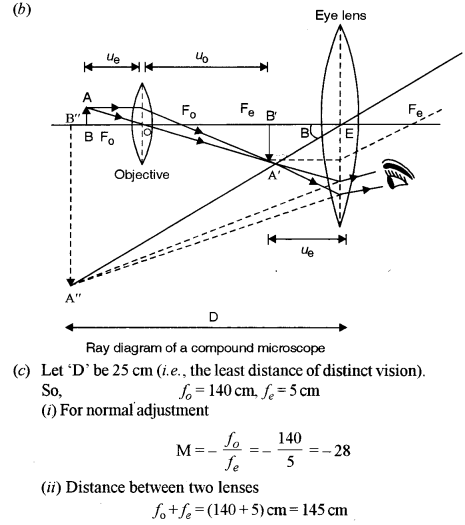Section-C

Question 8.
(a) State two important conclusions that can be drawn from Millikan’s oil drop experiment to determine the charge on an electron. 

(b) A monochromatic source of light emits light of wavelength 198 nm. Calculate: 
(i) energy of each photon;
(ii) momentum of the photon.

(c) (i) Name a series of lines of hydrogen spectrum which lies in : 

• Visible region.
• Ultraviolet region.

(ii) Write Bohr’s formula to calculate wavelength (λ,) of visible light, emitted by hydrogen, and explain the meaning of each and every symbol used.
(a) Important conclusions of Millikan’s oil drop method:
(i) The experiment established the quantum nature of the electric charge i. e.,Q = ne, where n is an integer.
(ii) it also helps us to find the mass of the electrons and its radius.Question 9.
(a) Starting with the law of radioactive disintegration, show that: $$\mathbf{N}=\mathbf{N}_{0} \mathbf{e}^{-\lambda \mathbf{t}}$$, where the terms have their usual meaning. 

(b) What is meant by Pair Production ? Explain with the help of an example and a balanced equation. 

(c) An X ray tube is operated at a tube potential of 40,000 V. Calculate: 
(i) Kinetic energy of an electron emitted by the filament when it reaches the target/anode.
(ii) Wavelengths of all the X rays emitted by the X ray tube.
The rate of decay of radioactive atoms at any instant is proportional to the number of atoms present at that instant.
Let ‘N’ be no. of atoms present in radioactive substance at any instant ‘t’. Let dN be the no. that disintegrates in a short interval ‘dt’. Then, the rate of disintegration -dN/dt is proportional to N,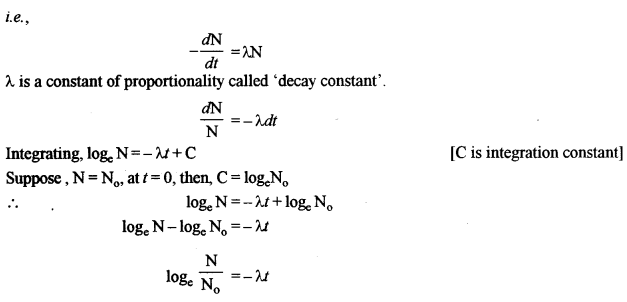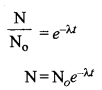(b) When an energetic y-ray photon falls on a heavy substance, it is absorbed by some nucleus of the substance and its energy gives rise to the production of an electron and a positron. This phenomenon in which energy is converted into mass is called pair production. It is an example of materialization of energy.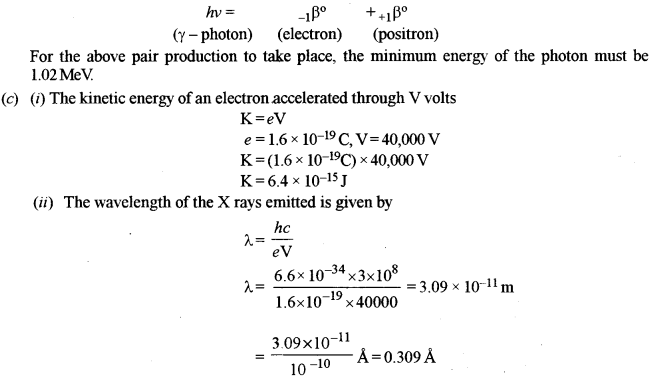Question 10.
(a) (i) In the following nuclear reaction, calculate the energy released in MeV:(ii) What is the name of this reaction ?

(b) What is meant by the terms : 
(i) a full wave rectifier?
(ii) an amplifier?
(iii) oscillator?

(c) Using several NAND gates, how can you obtain an AND gate ? Draw a labelled diagram in support of your answer.